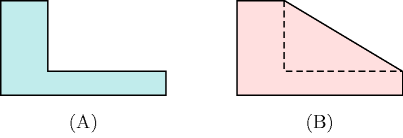### 3.7.64. Convex hull relaxation

Given a non-convex set $𝒮$, $ℛ$ is a convex outer approximation of $𝒮$ if:

• $ℛ$ is convex,

• If $s\in 𝒮$, then $s\in ℛ$.

Given a non-convex set $𝒮$, $ℛ$ is the convex hull of $𝒮$ if:

• $ℛ$ is a convex outer approximation of $𝒮$,

• For every $𝒯$ where $𝒯$ is a convex outer approximation of $𝒮$, $ℛ\subseteq 𝒯$.

Part (A) of Figure 3.7.17 depicts a non-convex set, while part (B) gives its corresponding convex hull.

##### Figure 3.7.17. (B) Convex hull of a (A) non-convex setWithin the context of linear programming the convex hull relaxation of a non-convex set $𝒮$ corresponds to the set of linear constraints characterising the convex hull of $𝒮$.